Check the below Online Education NCERT MCQ Questions for Class 10 Maths Chapter 10 Circles with Answers Pdf free download. MCQ Questions for Class 10 Maths with Answers were prepared based on the latest exam pattern. We have provided Circles Class 10 Maths MCQs Questions with Answers to help students understand the concept very well. https://ncertmcq.com/mcq-questions-for-class-10-maths-with-answers/

Students can also refer to NCERT Solutions for Class 10 Maths Chapter 10 Circles for better exam preparation and score more marks.

## Circles Class 10 MCQs Questions with Answers

Circles Class 10 MCQ Question 1.
If radii of two concentric circles are 4 cm and 5 cm, then the length of each chord of one circle which is tangent to the other circle is
(a) 3 cm
(b) 6 cm
(c) 9 cm
(d) 1 cm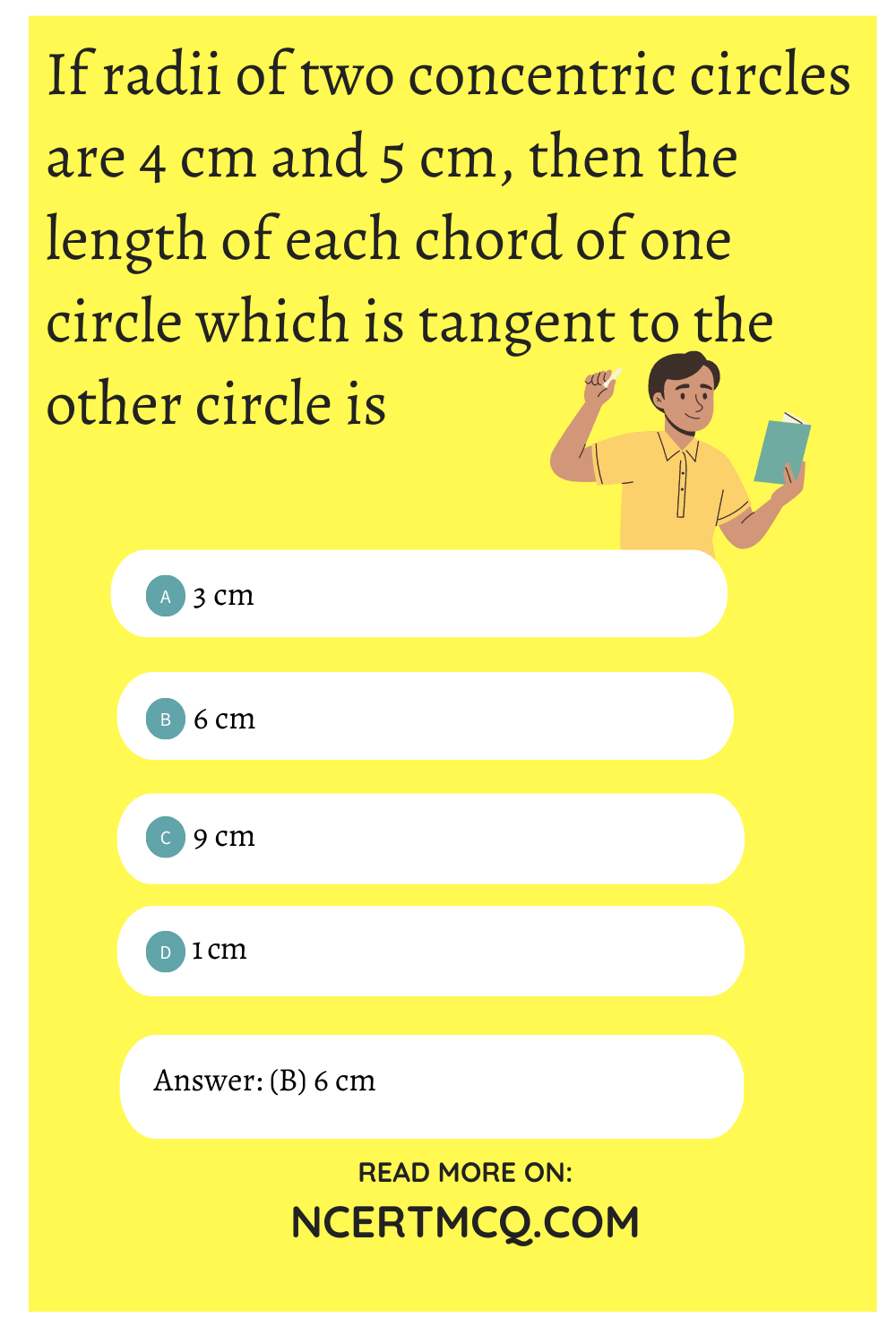Circles Class 10 MCQ With Answers Question 2.
In Fig., if ∠AOB = 125°, then ∠COD is equal to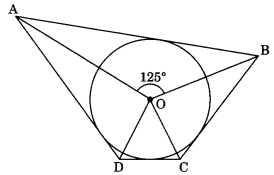(a) 62.5°
(b) 45°
(c) 35°
(d) 55°

Circle Class 10 MCQ Question 3.
If Fig., AB is a chord of the circle and AOC is its diameter such that ∠ACB = 50°. If AT is the tangent to the circle at the point A, the ∠BAT is equal to(a) 65°
(b) 60°
(c) 50°
(d) 40°

Circle MCQ Questions Class 10 Question 4.
From a point P which is at a distance of 13 cm from the point O of a circle of radius 5 cm, the pair of tangents PQ and PR to the circle are drawn. Then the area of the quadrilateral PQOR is
(a) 60 cm²
(b) 65 cm²
(c) 30 cm²
(d) 32.5 cm²

Class 10 Circles MCQ Question 5.
At one end A of a diameter AB of a circle of radius 5 cm, tangent XAY is drawn to the circle. The length of the chord CD parallel to XY and at a distance 8 cm from A is
(a) 4 cm
(b) 5 cm
(c) 6 cm
(d) 8 cm

Circles MCQ Class 10 Question 6.
In Fig., AT is a tangent to the circle with centre O such that OT = 4 cm and ∠OTA = 30°. Then AT is equal to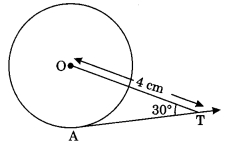(a) 4 cm
(b) 2 cm
(c) 2√3 cm
(d) 4√3 cm

MCQ On Circles Class 10 Question 7.
In Fig., if O is the centre of a circle PQ is a chord and the tangent PR at P makes an angle of 50° with PQ, then ∠POQ is equal to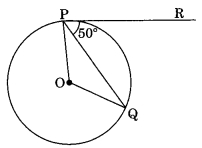(a) 100°
(b) 80°
(c) 90°
(d) 75°

Class 10 Maths Chapter 10 MCQ Question 8.
In Fig., if PA and PB are tangents to the circle with centre 0 such that ∠APB = 50°, then ∠AOB is equal to(a) 25°
(b) 130°
(c) 100°
(d) 50°

MCQ Of Circles Class 10 Question 9.
If two tangents inclined at an angle 60° are drawn to a circle of radius 3 cm the length of each tangent is equal to
(a) $$\frac{3}{2}$$√3 cm
(b) 6 cm
(c) 3 cm
(d) 3√3 cm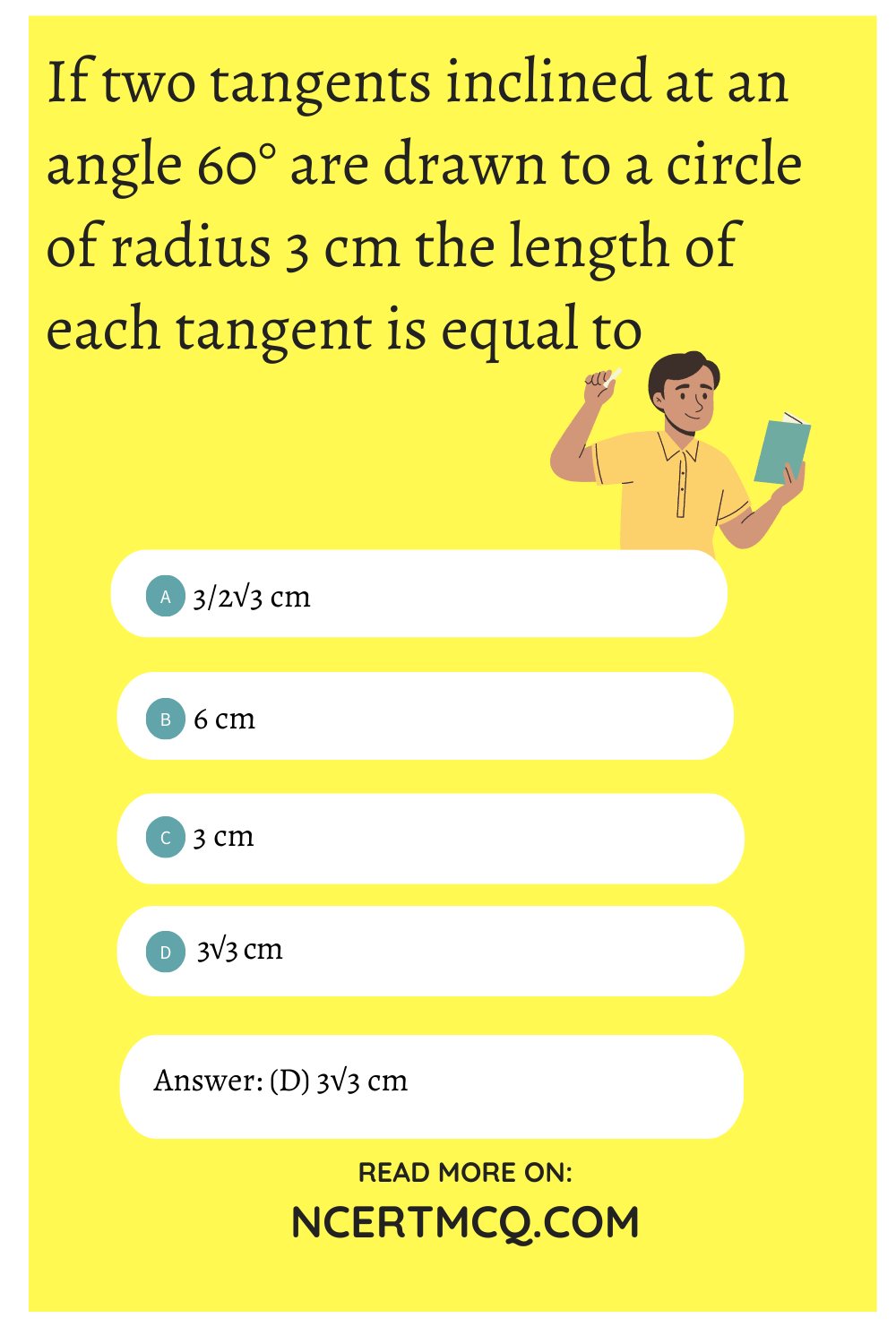Chapter 10 Maths Class 10 MCQ Question 10.
In Fig., if PQR is the tangent to a circle at Q whose centre is O, AB is a chord parallel to PR and ∠BQR = 70°, then ∠AQB is equal to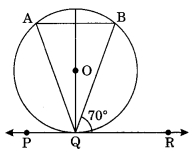(a) 20°
(b) 40°
(c) 35°
(d) 45°